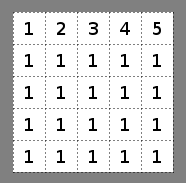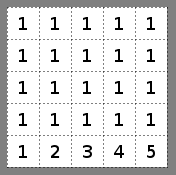# 2d Array on mesh presented reversed or backwards? FIXED

Good afternoon all,

I seem to have my thoughts in a knot. I wish to present my mesh in the same fashion as if you were to read a 2D array. I have the script built so as if it can read in my 2d Array and present the data via JSON scripts, but the mesh doesn’t properly present the data given.As your can see, on the left is the required output, while on the right is the result due to my scripting.

If someone could help me out, that would be great. My mind seems to be out of gas on this problem.

``````private void BuildMesh()
{
int numTiles = m_RootObject.width * m_RootObject.height;
int numTris = numTiles * 2;

int vsize_x = m_RootObject.width + 1;
int vsize_z = m_RootObject.height + 1;
int numVerts = vsize_x * vsize_z;

// Generate the mesh data
Vector3[] vertices = new Vector3[ numVerts ];
Vector3[] normals = new Vector3[numVerts];
Vector2[] uv = new Vector2[numVerts];

int[] triangles = new int[ numTris * 3 ];

int x, z;
for(z=0; z < vsize_z; z++)
{
for(x=0; x < vsize_x; x++)
{
vertices[ z * vsize_x + x ] = new Vector3( x, 0, z );
normals[ z * vsize_x + x ] = Vector3.up;
uv[ z * vsize_x + x ] = new Vector2( (float)x / (m_RootObject.width), (float)z / (m_RootObject.height) );
}
}

for(z=0; z < m_RootObject.height; z++)
{
for(x=0; x < m_RootObject.width; x++)
{
int squareIndex = z * m_RootObject.width + x;
int triOffset = squareIndex * 6;
triangles[triOffset + 0] = z * vsize_x + x + 0;
triangles[triOffset + 1] = z * vsize_x + x + vsize_x + 0;
triangles[triOffset + 2] = z * vsize_x + x + vsize_x + 1;

triangles[triOffset + 3] = z * vsize_x + x + 0;
triangles[triOffset + 4] = z * vsize_x + x + vsize_x + 1;
triangles[triOffset + 5] = z * vsize_x + x + 1;
}
}

// Create a new Mesh and populate with the data
Mesh mesh = new Mesh();
mesh.vertices = vertices;
mesh.triangles = triangles;
mesh.normals = normals;
mesh.uv = uv;

// Assign our mesh to our filter/renderer/collider
MeshFilter mesh_filter = GetComponent<MeshFilter>();
MeshCollider mesh_collider = GetComponent<MeshCollider>();

mesh_filter.mesh = mesh;
mesh_collider.sharedMesh = mesh;
}
``````

Here is some more scripts, hopefully it can help find the issue.

``````private void BuildTexture()
{
int texWidth = m_RootObject.width * 32;
int texHeight = m_RootObject.height * 32;
Texture2D texture = new Texture2D(texWidth, texHeight);

Color[][] tiles = ChopUpTiles();

//m_RootObject.layers.data.Reverse();
Debug.Log(m_RootObject.width);
int g = 0;
for(int y=0; y < m_RootObject.height; y++)
{
for(int x=0; x < m_RootObject.width; x++)
{
Color[] p = tiles[ (m_RootObject.layers.data[g]-1 == -1) ? 0 : m_RootObject.layers.data[g]-1];
texture.SetPixels(x*32, y*32, 32, 32, p);
g++;
}
}

texture.filterMode = FilterMode.Point;
texture.wrapMode = TextureWrapMode.Clamp;
texture.Apply();

MeshRenderer mesh_renderer = GetComponent<MeshRenderer>();
mesh_renderer.sharedMaterials.mainTexture = texture;
}

Color[][] ChopUpTiles()
{
int numTilesPerRow = terrainTiles.width / 32;
int numRows = terrainTiles.height / 32;

Color[][] tiles = new Color[numTilesPerRow*numRows][];

for(int y=0; y<numRows; y++)
{
for(int x=0; x<numTilesPerRow; x++)
{
tiles[y*numTilesPerRow + x] = terrainTiles.GetPixels( x*32, y*32, 32, 32 );
}
}

return tiles;
}
``````

Fixed my issue, only took me all day.

If anyone is interested, here is the pastebin code.